Jake's Math LessonsThe top of a ladder slides down a vertical wall at a rate of 0.15 m/s . At the moment when the bottom of the ladder is 3 m from the wall, it slides away from the wall at a rate of 0.2 m/s . How long is the ladder?

This is a fairly common example of a related rates problem and a common application of derivatives and implicit differentiation . I’m sure you may have come across a related rates ladder problem like this. If I can offer one piece of advice for this type of problem it’d be this: don’t use this ladder, it always falls…

Alright, bad jokes aside, this is going to follow the same 4 steps as all the other related rates problems I’ve done. If you’d rather watch a video, then check out my video below. But otherwise, let’s jump into it with the usual process!

## 1. Draw a sketch

As always, we’ll start by drawing a quick sketch of all of the information that is being described in the problem. To do this we should first think about what information we have. First of all, we need to think about the shape that’s being formed with the ladder.

Since the ladder is standing on the ground and leaning up against a vertical wall, we can say that a triangle would be formed by the 3 objects in the problem. More specifically we know that the vertical wall forms a 90 degree angle with the ground. Therefore, the triangle formed by the ground, the wall, and the ladder would be a right triangle .On top of this, the problem also gives us a few pieces of information about the dimensions of the triangle and how they are changing. It actually tells us about how fast the ladder is moving, but since the ladder is what forms the triangle, we can deduce how the dimensions of the triangle are changing.

We are given 3 pieces of information about the position of the ladder as well as how the ladder is moving at the specific instant we are looking at.

• Bottom of the ladder is 3 m away from the wall.
• Top of the ladder is moving down the wall at a rate of 0.15 m/s .
• Bottom of the ladder is moving away from the wall at a rate of 0.2 m/s .

Adding these labels to our drawing from above would give us something like this: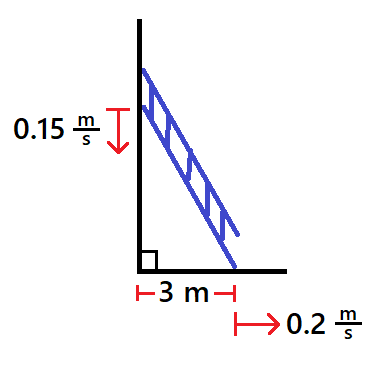This sketch gives us a pretty good idea of what is going on in this problem. Not only that, but we will be able to use this to get an idea of what kind of equation we will need to come up with.

## 2. Come up with your equation

Before we come up with our equation we want to sort through the information we are given and asked to find. This is important because we need to decide what measurements and variables we want in the equation.

## What are we looking for?

The question asks us to find the length of the ladder. Therefore, we will need to find the length of the hypotenuse of the triangle in our drawing. Because of this we want to be sure to include the hypotenuse of our triangle in our equation.

## What do we know about?

Looking back up at our labeled drawing, you can see that we really only have information about the bottom and side of our triangle. We know the length of the bottom side of the triangle and the rate of change of this side.

And we were also given information about the rate of change of the left side of the triangle. But remember that our equation in this step cannot include rates of change . Instead, the fact that we know this rate of change tells us that we can use the left side length of the triangle in our equation, not its rate of change.

We aren’t given any information about the angles in the triangle other than the fact that it’s a right triangle. As a result, we probably don’t want our equation to involve the angles of this triangle.

Since we know that our equation either needs to include, or can include the lengths of the sides of the triangle we should label them. Let’s go back to our drawing and label the side of the triangle. You can label them whatever you’d like, but I’ll go with x , y , and z .## Putting it into an equation

At this point we’ve figured out that we need an equation that related the sides of a right triangle.

What do you know about the relationship between the sides of a right triangle, but neither of the other two angles?

You’re probably thinking Pythagorean Theorem. If you are, you’re right! Pythagorean Theorem tells us that the square of the hypotenuse is equal to the sum of the squares of the other two sides of a right triangle. Remember this can only be applied to the sides of a right triangle, so noticing that is actually very important. In other words we know $$z^2=x^2+y^2.$$

## 3. Implicit differentiation

Now that we have come up with our equation, we need to apply implicit differentiation to take the derivative of both sides of our equation with respect to time.

$$\frac{d}{dt} \Big[ z^2 = x^2 + y^2 \Big]$$

Before we do this though I want to point something out. Let’s look at each of the letters in this equation and consider how we need to treat them when we differentiate with respect to time.$z^2$

So when we take the derivative of z with respect to time, the derivative will be 0 . The derivative of any constant is 0 .$x^2$

## Back to the derivative

Knowing how to treat each letter in our equation, let’s go ahead and take the derivative of both sides with respect to time.

$$\frac{d}{dt} \Big[ z^2 = x^2 + y^2 \Big]$$ $$0 = 2x \frac{dx}{dt} + 2y \frac{dy}{dt}$$

## 4. Solve for the desired rate of change

Now all we need to do is plug in all of the information we have and solve for the right variable. However, this one is a little weird. The reason I say this is that we are actually not looking for a rate of change.

Remember the question told us to find the length of the ladder. Which means we need to find the value of z . The differential equation we just ended up with doesn’t even have a z in it, so how can we use it to find z ?

Well, we’re actually going to need to go back to our original equation. $$z^2=x^2+y^2$$ We know the value of x based on information we were given, but we don’t know the value of y yet. If we could figure out what y was, then we could use this equation, plug in the value for x and y , then solve for z .

## How do we find y ?

This is what we will use our differential equation from the previous step for. That equation has a y in it, and we know the value of all the other variables.$\mathbf{x=3}$

## Plugging it all into our equation

Knowing all of the values in our equation aside from y , we can plug these in and solve for y .

$$0 = 2x \frac{dx}{dt} + 2y \frac{dy}{dt}$$ $$0 = 2(3)(0.2) + 2y(-0.15)$$ $$0=1.2-0.3y$$ $$0.3y=1.2$$ $$y=4$$

Now that we know x and y , we can plug them back into our original equation and solve for z .

$$z^2=x^2+y^2$$ $$z^2=(3)^2+(4)^2$$ $$z^2=25$$ $$z=5$$

So the ladder must be 5 m long!

Notify me of follow-up comments by email.

Notify me of new posts by email.

• 4.1 Related Rates
• Introduction
• 1.1 Review of Functions
• 1.2 Basic Classes of Functions
• 1.3 Trigonometric Functions
• 1.4 Inverse Functions
• 1.5 Exponential and Logarithmic Functions
• Key Equations
• Key Concepts
• Review Exercises
• 2.1 A Preview of Calculus
• 2.2 The Limit of a Function
• 2.3 The Limit Laws
• 2.4 Continuity
• 2.5 The Precise Definition of a Limit
• 3.1 Defining the Derivative
• 3.2 The Derivative as a Function
• 3.3 Differentiation Rules
• 3.4 Derivatives as Rates of Change
• 3.5 Derivatives of Trigonometric Functions
• 3.6 The Chain Rule
• 3.7 Derivatives of Inverse Functions
• 3.8 Implicit Differentiation
• 3.9 Derivatives of Exponential and Logarithmic Functions
• 4.2 Linear Approximations and Differentials
• 4.3 Maxima and Minima
• 4.4 The Mean Value Theorem
• 4.5 Derivatives and the Shape of a Graph
• 4.6 Limits at Infinity and Asymptotes
• 4.7 Applied Optimization Problems
• 4.8 L’Hôpital’s Rule
• 4.9 Newton’s Method
• 4.10 Antiderivatives
• 5.1 Approximating Areas
• 5.2 The Definite Integral
• 5.3 The Fundamental Theorem of Calculus
• 5.4 Integration Formulas and the Net Change Theorem
• 5.5 Substitution
• 5.6 Integrals Involving Exponential and Logarithmic Functions
• 5.7 Integrals Resulting in Inverse Trigonometric Functions
• 6.1 Areas between Curves
• 6.2 Determining Volumes by Slicing
• 6.3 Volumes of Revolution: Cylindrical Shells
• 6.4 Arc Length of a Curve and Surface Area
• 6.5 Physical Applications
• 6.6 Moments and Centers of Mass
• 6.7 Integrals, Exponential Functions, and Logarithms
• 6.8 Exponential Growth and Decay
• 6.9 Calculus of the Hyperbolic Functions
• A | Table of Integrals
• B | Table of Derivatives
• C | Review of Pre-Calculus

## Learning Objectives

• 4.1.1 Express changing quantities in terms of derivatives.
• 4.1.2 Find relationships among the derivatives in a given problem.
• 4.1.3 Use the chain rule to find the rate of change of one quantity that depends on the rate of change of other quantities.

We have seen that for quantities that are changing over time, the rates at which these quantities change are given by derivatives. If two related quantities are changing over time, the rates at which the quantities change are related. For example, if a balloon is being filled with air, both the radius of the balloon and the volume of the balloon are increasing. In this section, we consider several problems in which two or more related quantities are changing and we study how to determine the relationship between the rates of change of these quantities.

## Setting up Related-Rates Problems

In many real-world applications, related quantities are changing with respect to time. For example, if we consider the balloon example again, we can say that the rate of change in the volume, V , V , is related to the rate of change in the radius, r . r . In this case, we say that d V d t d V d t and d r d t d r d t are related rates because V is related to r . Here we study several examples of related quantities that are changing with respect to time and we look at how to calculate one rate of change given another rate of change.

## Example 4.1

Inflating a balloon.

A spherical balloon is being filled with air at the constant rate of 2 cm 3 / sec 2 cm 3 / sec ( Figure 4.2 ). How fast is the radius increasing when the radius is 3 cm ? 3 cm ?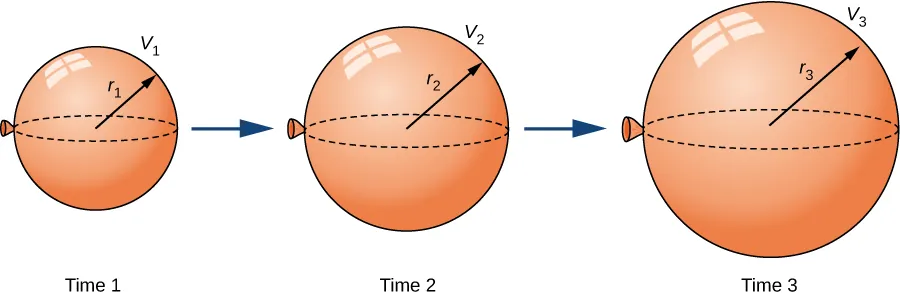The volume of a sphere of radius r r centimeters is

Since the balloon is being filled with air, both the volume and the radius are functions of time. Therefore, t t seconds after beginning to fill the balloon with air, the volume of air in the balloon is

Differentiating both sides of this equation with respect to time and applying the chain rule, we see that the rate of change in the volume is related to the rate of change in the radius by the equation

The balloon is being filled with air at the constant rate of 2 cm 3 /sec, so V ′ ( t ) = 2 cm 3 / sec . V ′ ( t ) = 2 cm 3 / sec . Therefore,

which implies

When the radius r = 3 cm, r = 3 cm,

## Checkpoint 4.1

What is the instantaneous rate of change of the radius when r = 6 cm ? r = 6 cm ?

Before looking at other examples, let’s outline the problem-solving strategy we will be using to solve related-rates problems.

## Problem-Solving Strategy

Problem-solving strategy: solving a related-rates problem.

• Assign symbols to all variables involved in the problem. Draw a figure if applicable.
• State, in terms of the variables, the information that is given and the rate to be determined.
• Find an equation relating the variables introduced in step 1.
• Using the chain rule, differentiate both sides of the equation found in step 3 with respect to the independent variable. This new equation will relate the derivatives.
• Substitute all known values into the equation from step 4, then solve for the unknown rate of change.

Note that when solving a related-rates problem, it is crucial not to substitute known values too soon. For example, if the value for a changing quantity is substituted into an equation before both sides of the equation are differentiated, then that quantity will behave as a constant and its derivative will not appear in the new equation found in step 4. We examine this potential error in the following example.

## Examples of the Process

Let’s now implement the strategy just described to solve several related-rates problems. The first example involves a plane flying overhead. The relationship we are studying is between the speed of the plane and the rate at which the distance between the plane and a person on the ground is changing.

## Example 4.2

An airplane flying at a constant elevation.

An airplane is flying overhead at a constant elevation of 4000 ft . 4000 ft . A man is viewing the plane from a position 3000 ft 3000 ft from the base of a radio tower. The airplane is flying horizontally away from the man. If the plane is flying at the rate of 600 ft/sec , 600 ft/sec , at what rate is the distance between the man and the plane increasing when the plane passes over the radio tower?

Step 1. Draw a picture, introducing variables to represent the different quantities involved.As shown, x x denotes the distance between the man and the position on the ground directly below the airplane. The variable s s denotes the distance between the man and the plane. Note that both x x and s s are functions of time. We do not introduce a variable for the height of the plane because it remains at a constant elevation of 4000 ft . 4000 ft . Since an object’s height above the ground is measured as the shortest distance between the object and the ground, the line segment of length 4000 ft is perpendicular to the line segment of length x x feet, creating a right triangle.

Step 2. Since x x denotes the horizontal distance between the man and the point on the ground below the plane, d x / d t d x / d t represents the speed of the plane. We are told the speed of the plane is 600 ft/sec. Therefore, d x d t = 600 d x d t = 600 ft/sec. Since we are asked to find the rate of change in the distance between the man and the plane when the plane is directly above the radio tower, we need to find d s / d t d s / d t when x = 3000 ft . x = 3000 ft .

Step 3. From the figure, we can use the Pythagorean theorem to write an equation relating x x and s : s :

Step 4. Differentiating this equation with respect to time and using the fact that the derivative of a constant is zero, we arrive at the equation

Step 5. Find the rate at which the distance between the man and the plane is increasing when the plane is directly over the radio tower. That is, find d s d t d s d t when x = 3000 ft . x = 3000 ft . Since the speed of the plane is 600 ft/sec , 600 ft/sec , we know that d x d t = 600 ft/sec . d x d t = 600 ft/sec . We are not given an explicit value for s ; s ; however, since we are trying to find d s d t d s d t when x = 3000 ft , x = 3000 ft , we can use the Pythagorean theorem to determine the distance s s when x = 3000 x = 3000 and the height is 4000 ft . 4000 ft . Solving the equation

for s , s , we have s = 5000 ft s = 5000 ft at the time of interest. Using these values, we conclude that d s / d t d s / d t is a solution of the equation

Note : When solving related-rates problems, it is important not to substitute values for the variables too soon. For example, in step 3, we related the variable quantities x ( t ) x ( t ) and s ( t ) s ( t ) by the equation

Since the plane remains at a constant height, it is not necessary to introduce a variable for the height, and we are allowed to use the constant 4000 to denote that quantity. However, the other two quantities are changing. If we mistakenly substituted x ( t ) = 3000 x ( t ) = 3000 into the equation before differentiating, our equation would have been

After differentiating, our equation would become

As a result, we would incorrectly conclude that d s d t = 0 . d s d t = 0 .

## Checkpoint 4.2

What is the speed of the plane if the distance between the person and the plane is increasing at the rate of 300 ft/sec ? 300 ft/sec ?

We now return to the problem involving the rocket launch from the beginning of the chapter.

## Example 4.3

Chapter opener: a rocket launch.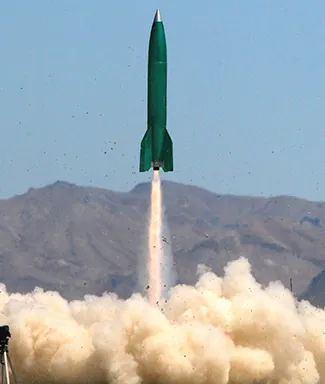A rocket is launched so that it rises vertically. A camera is positioned 5000 ft 5000 ft from the launch pad. When the rocket is 1000 ft 1000 ft above the launch pad, its velocity is 600 ft/sec . 600 ft/sec . Find the necessary rate of change of the camera’s angle as a function of time so that it stays focused on the rocket.

Step 1. Draw a picture introducing the variables.Let h h denote the height of the rocket above the launch pad and θ θ be the angle between the camera lens and the ground.

Step 2. We are trying to find the rate of change in the angle of the camera with respect to time when the rocket is 1000 ft off the ground. That is, we need to find d θ d t d θ d t when h = 1000 ft . h = 1000 ft . At that time, we know the velocity of the rocket is d h d t = 600 ft/sec . d h d t = 600 ft/sec .

Step 3. Now we need to find an equation relating the two quantities that are changing with respect to time: h h and θ . θ . How can we create such an equation? Using the fact that we have drawn a right triangle, it is natural to think about trigonometric functions. Recall that tan θ tan θ is the ratio of the length of the opposite side of the triangle to the length of the adjacent side. Thus, we have

This gives us the equation

Step 4. Differentiating this equation with respect to time t , t , we obtain

Step 5. We want to find d θ d t d θ d t when h = 1000 ft . h = 1000 ft . At this time, we know that d h d t = 600 ft/sec . d h d t = 600 ft/sec . We need to determine sec 2 θ . sec 2 θ . Recall that sec θ sec θ is the ratio of the length of the hypotenuse to the length of the adjacent side. We know the length of the adjacent side is 5000 ft . 5000 ft . To determine the length of the hypotenuse, we use the Pythagorean theorem, where the length of one leg is 5000 ft , 5000 ft , the length of the other leg is h = 1000 ft , h = 1000 ft , and the length of the hypotenuse is c c feet as shown in the following figure.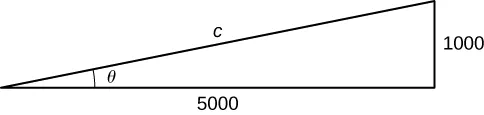We see that

and we conclude that the hypotenuse is

Therefore, when h = 1000 , h = 1000 , we have

Recall from step 4 that the equation relating d θ d t d θ d t to our known values is

When h = 1000 ft , h = 1000 ft , we know that d h d t = 600 ft/sec d h d t = 600 ft/sec and sec 2 θ = 26 25 . sec 2 θ = 26 25 . Substituting these values into the previous equation, we arrive at the equation

Therefore, d θ d t = 3 26 rad/sec . d θ d t = 3 26 rad/sec .

## Checkpoint 4.3

What rate of change is necessary for the elevation angle of the camera if the camera is placed on the ground at a distance of 4000 ft 4000 ft from the launch pad and the velocity of the rocket is 500 ft/sec when the rocket is 2000 ft 2000 ft off the ground?

In the next example, we consider water draining from a cone-shaped funnel. We compare the rate at which the level of water in the cone is decreasing with the rate at which the volume of water is decreasing.

## Example 4.4

Water draining from a funnel.

Water is draining from the bottom of a cone-shaped funnel at the rate of 0.0 3 ft 3 /sec . 0.0 3 ft 3 /sec . The height of the funnel is 2 ft and the radius at the top of the funnel is 1 ft . 1 ft . At what rate is the height of the water in the funnel changing when the height of the water is 1 2 ft ? 1 2 ft ?

Step 1: Draw a picture introducing the variables.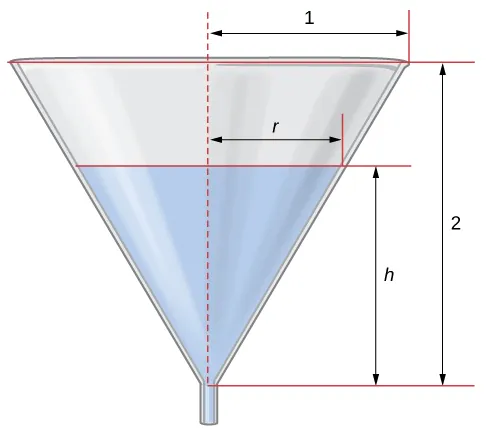Let h h denote the height of the water in the funnel, r r denote the radius of the water at its surface, and V V denote the volume of the water.

Step 2: We need to determine d h d t d h d t when h = 1 2 ft . h = 1 2 ft . We know that d V d t = −0.03 ft 3 /sec . d V d t = −0.03 ft 3 /sec .

Step 3: The volume of water in the cone is

From the figure, we see that we have similar triangles. Therefore, the ratio of the sides in the two triangles is the same. Therefore, r h = 1 2 r h = 1 2 or r = h 2 . r = h 2 . Using this fact, the equation for volume can be simplified to

Step 4: Applying the chain rule while differentiating both sides of this equation with respect to time t , t , we obtain

Step 5: We want to find d h d t d h d t when h = 1 2 ft . h = 1 2 ft . Since water is leaving at the rate of 0.0 3 ft 3 /sec , 0.0 3 ft 3 /sec , we know that d V d t = −0.03 ft 3 /sec . d V d t = −0.03 ft 3 /sec . Therefore,

It follows that

## Checkpoint 4.4

At what rate is the height of the water changing when the height of the water is 1 4 ft ? 1 4 ft ?

## Section 4.1 Exercises

For the following exercises, find the quantities for the given equation.

Find d y d t d y d t at x = 1 x = 1 and y = x 2 + 3 y = x 2 + 3 if d x d t = 4 . d x d t = 4 .

Find d x d t d x d t at x = −2 x = −2 and y = 2 x 2 + 1 y = 2 x 2 + 1 if d y d t = −1 . d y d t = −1 .

Find d z d t d z d t at ( x , y ) = ( 1 , 3 ) ( x , y ) = ( 1 , 3 ) and z 2 = x 2 + y 2 z 2 = x 2 + y 2 if d x d t = 4 d x d t = 4 and d y d t = 3 . d y d t = 3 .

For the following exercises, sketch the situation if necessary and used related rates to solve for the quantities.

[T] If two electrical resistors are connected in parallel, the total resistance (measured in ohms, denoted by the Greek capital letter omega, Ω ) Ω ) is given by the equation 1 R = 1 R 1 + 1 R 2 . 1 R = 1 R 1 + 1 R 2 . If R 1 R 1 is increasing at a rate of 0.5 Ω / min 0.5 Ω / min and R 2 R 2 decreases at a rate of 1.1 Ω/min , 1.1 Ω/min , at what rate does the total resistance change when R 1 = 20 Ω R 1 = 20 Ω and R 2 = 50 Ω R 2 = 50 Ω ?

A 10-ft ladder is leaning against a wall. If the top of the ladder slides down the wall at a rate of 2 ft/sec, how fast is the bottom moving along the ground when the bottom of the ladder is 5 ft from the wall?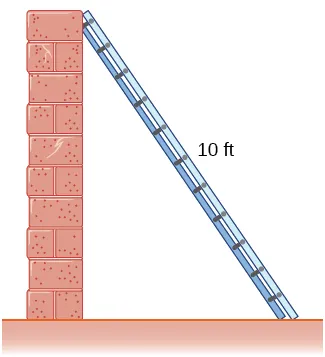A 25-ft ladder is leaning against a wall. If we push the ladder toward the wall at a rate of 1 ft/sec, and the bottom of the ladder is initially 20 ft 20 ft away from the wall, how fast does the ladder move up the wall 5 sec 5 sec after we start pushing?

Two airplanes are flying in the air at the same height: airplane A is flying east at 250 mi/h and airplane B is flying north at 300 mi/h . 300 mi/h . If they are both heading to the same airport, located 30 miles east of airplane A and 40 miles north of airplane B , at what rate is the distance between the airplanes changing?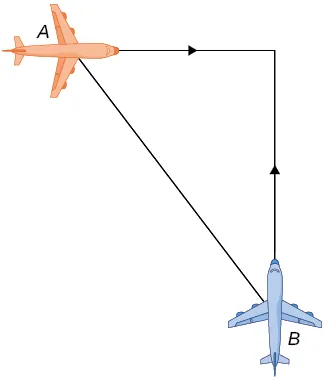You and a friend are riding your bikes to a restaurant that you think is east; your friend thinks the restaurant is north. You both leave from the same point, with you riding at 16 mph east and your friend riding 12 mph 12 mph north. After you traveled 4 mi, 4 mi, at what rate is the distance between you changing?

Two buses are driving along parallel freeways that are 5 mi 5 mi apart, one heading east and the other heading west. Assuming that each bus drives a constant 55 mph, 55 mph, find the rate at which the distance between the buses is changing when they are 13 mi 13 mi apart, heading toward each other.

A 6-ft-tall person walks away from a 10-ft lamppost at a constant rate of 3 ft/sec . 3 ft/sec . What is the rate that the tip of the shadow moves away from the pole when the person is 10 ft 10 ft away from the pole?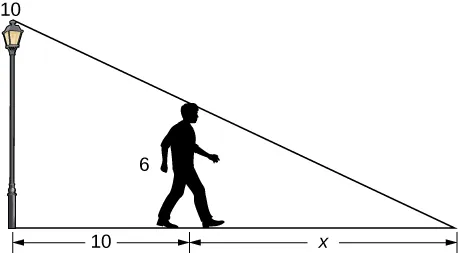Using the previous problem, what is the rate at which the tip of the shadow moves away from the person when the person is 10 ft from the pole?

A 5-ft-tall person walks toward a wall at a rate of 2 ft/sec. A spotlight is located on the ground 40 ft from the wall. How fast does the height of the person’s shadow on the wall change when the person is 10 ft from the wall?

Using the previous problem, what is the rate at which the shadow changes when the person is 10 ft from the wall, if the person is walking away from the wall at a rate of 2 ft/sec?

A helicopter starting on the ground is rising directly into the air at a rate of 25 ft/sec. You are running on the ground starting directly under the helicopter at a rate of 10 ft/sec. Find the rate of change of the distance between the helicopter and yourself after 5 sec.

Using the previous problem, what is the rate at which the distance between you and the helicopter is changing when the helicopter has risen to a height of 60 ft in the air, assuming that, initially, it was 30 ft above you?

For the following exercises, draw and label diagrams to help solve the related-rates problems.

The side of a cube increases at a rate of 1 2 1 2 m/sec. Find the rate at which the volume of the cube increases when the side of the cube is 4 m.

The volume of a cube decreases at a rate of 10 m 3 /s. Find the rate at which the side of the cube changes when the side of the cube is 2 m.

The radius of a circle increases at a rate of 2 2 m/sec. Find the rate at which the area of the circle increases when the radius is 5 m.

The radius of a sphere decreases at a rate of 3 3 m/sec. Find the rate at which the surface area decreases when the radius is 10 m.

The radius of a sphere increases at a rate of 1 1 m/sec. Find the rate at which the volume increases when the radius is 20 20 m.

The radius of a sphere is increasing at a rate of 9 cm/sec. Find the radius of the sphere when the volume and the radius of the sphere are increasing at the same numerical rate.

The base of a triangle is shrinking at a rate of 1 cm/min and the height of the triangle is increasing at a rate of 5 cm/min. Find the rate at which the area of the triangle changes when the height is 22 cm and the base is 10 cm.

A triangle has two constant sides of length 3 ft and 5 ft. The angle between these two sides is increasing at a rate of 0.1 rad/sec. Find the rate at which the area of the triangle is changing when the angle between the two sides is π / 6 . π / 6 .

A triangle has a height that is increasing at a rate of 2 cm/sec and its area is increasing at a rate of 4 cm 2 /sec. Find the rate at which the base of the triangle is changing when the height of the triangle is 4 cm and the area is 20 cm 2 .

For the following exercises, consider a right cone that is leaking water. The dimensions of the conical tank are a height of 16 ft and a radius of 5 ft.

How fast does the depth of the water change when the water is 10 ft high if the cone leaks water at a rate of 10 ft 3 /min?

Find the rate at which the surface area of the water changes when the water is 10 ft high if the cone leaks water at a rate of 10 ft 3 /min.

If the water level is decreasing at a rate of 3 in/min when the depth of the water is 8 ft, determine the rate at which water is leaking out of the cone.

A vertical cylinder is leaking water at a rate of 1 ft 3 /sec. If the cylinder has a height of 10 ft and a radius of 1 ft, at what rate is the height of the water changing when the height is 6 ft?

A cylinder is leaking water but you are unable to determine at what rate. The cylinder has a height of 2 m and a radius of 2 m. Find the rate at which the water is leaking out of the cylinder if the rate at which the height is decreasing is 10 cm/min when the height is 1 m.

A trough has ends shaped like isosceles triangles, with width 3 m and height 4 m, and the trough is 10 m long. Water is being pumped into the trough at a rate of 5 m 3 /min . 5 m 3 /min . At what rate does the height of the water change when the water is 1 m deep?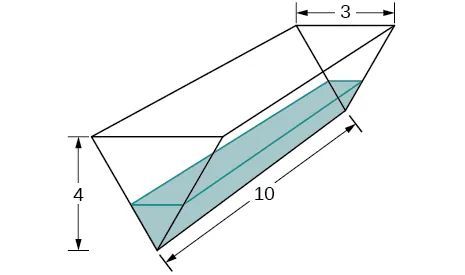A tank is shaped like an upside-down square pyramid, with base of 4 m by 4 m and a height of 12 m (see the following figure). How fast does the height increase when the water is 2 m deep if water is being pumped in at a rate of 2 3 2 3 m 3 /sec?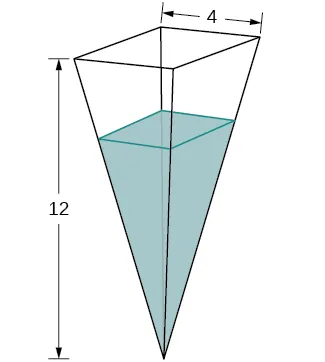For the following problems, consider a pool shaped like the bottom half of a sphere, that is being filled at a rate of 25 ft 3 /min. The radius of the pool is 10 ft. The formula for the volume of a partial hemisphere is V = πh 6 ( 3 r 2 + h 2 ) V = πh 6 ( 3 r 2 + h 2 ) where h h is the height of the water and r r is the radius of the water.

Find the rate at which the depth of the water is changing when the water has a depth of 5 ft.

Find the rate at which the depth of the water is changing when the water has a depth of 1 ft.

If the height is increasing at a rate of 1 in./min when the depth of the water is 2 ft, find the rate at which water is being pumped in.

Gravel is being unloaded from a truck and falls into a pile shaped like a cone at a rate of 10 ft 3 /min. The radius of the cone base is three times the height of the cone. Find the rate at which the height of the gravel changes when the pile has a height of 5 ft.

Using a similar setup from the preceding problem, find the rate at which the gravel is being unloaded if the pile is 5 ft high and the height is increasing at a rate of 4 in./min.

For the following exercises, draw the situations and solve the related-rate problems.

You are stationary on the ground and are watching a bird fly horizontally at a rate of 10 10 m/sec. The bird is located 40 m above your head. How fast does the angle of elevation change when the horizontal distance between you and the bird is 9 m?

You stand 40 ft from a bottle rocket on the ground and watch as it takes off vertically into the air at a rate of 20 ft/sec. Find the rate at which the angle of elevation changes when the rocket is 30 ft in the air.

A lighthouse, L , is on an island 4 mi away from the closest point, P , on the beach (see the following image). If the lighthouse light rotates clockwise at a constant rate of 10 revolutions/min, how fast does the beam of light move across the beach 2 mi away from the closest point on the beach?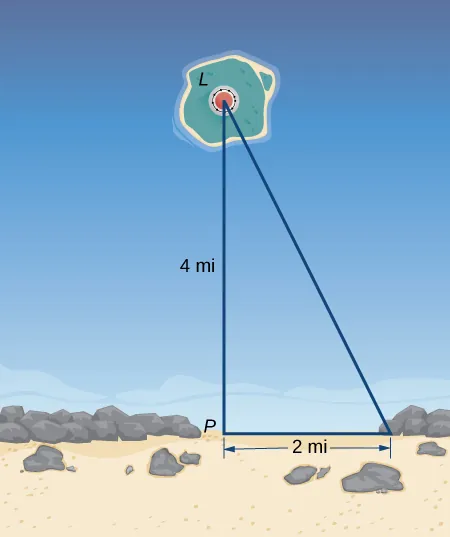Using the same setup as the previous problem, determine at what rate the beam of light moves across the beach 1 mi away from the closest point on the beach.

You are walking to a bus stop at a right-angle corner. You move north at a rate of 2 m/sec and are 20 m south of the intersection. The bus travels west at a rate of 10 m/sec away from the intersection – you have missed the bus! What is the rate at which the angle between you and the bus is changing when you are 20 m south of the intersection and the bus is 10 m west of the intersection?

For the following exercises, refer to the figure of baseball diamond, which has sides of 90 ft.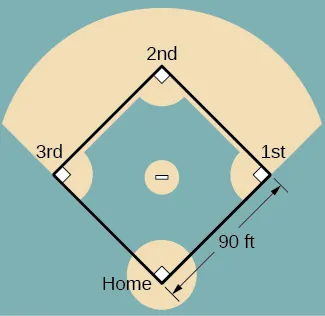[T] A batter hits a ball toward third base at 75 ft/sec and runs toward first base at a rate of 24 ft/sec. At what rate does the distance between the ball and the batter change when 2 sec have passed?

[T] A batter hits a ball toward second base at 80 ft/sec and runs toward first base at a rate of 30 ft/sec. At what rate does the distance between the ball and the batter change when the runner has covered one-third of the distance to first base? ( Hint : Recall the law of cosines.)

[T] A batter hits the ball and runs toward first base at a speed of 22 ft/sec. At what rate does the distance between the runner and second base change when the runner has run 30 ft?

[T] Runners start at first and second base. When the baseball is hit, the runner at first base runs at a speed of 18 ft/sec toward second base and the runner at second base runs at a speed of 20 ft/sec toward third base. How fast is the distance between runners changing 1 sec after the ball is hit?

As an Amazon Associate we earn from qualifying purchases.

Want to cite, share, or modify this book? This book uses the Creative Commons Attribution-NonCommercial-ShareAlike License and you must attribute OpenStax.

• Authors: Gilbert Strang, Edwin “Jed” Herman
• Publisher/website: OpenStax
• Book title: Calculus Volume 1
• Publication date: Mar 30, 2016
• Location: Houston, Texas
• Book URL: https://openstax.org/books/calculus-volume-1/pages/1-introduction
• Section URL: https://openstax.org/books/calculus-volume-1/pages/4-1-related-rates

© Jul 17, 2023 OpenStax. Textbook content produced by OpenStax is licensed under a Creative Commons Attribution-NonCommercial-ShareAlike License . The OpenStax name, OpenStax logo, OpenStax book covers, OpenStax CNX name, and OpenStax CNX logo are not subject to the Creative Commons license and may not be reproduced without the prior and express written consent of Rice University.

Calcworkshop

## Related Rates How To w/ 7+ Step-by-Step Examples!

// Last Updated: February 22, 2021 - Watch Video //

How do you solve related rates?Jenn, Founder Calcworkshop ® , 15+ Years Experience (Licensed & Certified Teacher)

Great question!

And that’s just what you’re going to learn how to do in today’s calculus lesson.

Let’s go!

## What Are Related Rates (Real Life Examples)

Have you ever watched a baseball player who is rounding third and heading for home and wondered if they had enough speed to make it before getting tagged out by the thrower?

Or have you ever watched a basketball player shoot a free-throw and speculate if the ball has enough height and distance?

Or perhaps you’ve listened to a guitar solo and contemplated the number of vibrations per second needed to make the guitar strings hum at the perfect pitch?

If you have, and even if you haven’t, all of these queries have something in common — something is changing with respect to time .

This is the idea behind related rates .

The baseball player’s distance to the home plate is changing with respect to the runner’s speed per second. The success of a free-throw is related to the ball’s projectile motion and the instantaneous rate of change of the height and distance traveled. And when a guitar string is plucked, the rate of the guitar string’s vibration (frequency) produces high or low pitches, which make the music we hear sound pleasing.## What Does It Mean If Two Rates Are Related

Let’s make sense of things using the image to the right.

Imagine a person is outside looking up into the sky and they spot an airplane that is flying at an altitude of 6 miles above the ground. Now, as the plane continues on its flight path, several things are changing with respect to each other.

The angle of elevation (theta), the line of sight (hypotenuse), as well as the horizontal distance are all changing as the plane flies overhead and with respect to time.

And that’s what it means for two or more rates to be related — as one rate changes, so does the other.Calculate the Speed of an Airplane

## How To Solve Related Rates Problems

We use the principles of problem-solving when solving related rates. The steps are as follows:

• Read the problem carefully and write down all the given information.
• Sketch and label a graph or diagram, if applicable.
• Find an equation that relates the unknown variable and known variable(s) by looking for geometric shapes, known formulas, ratios such as the Pythagorean theorem, area and volume formulas, or trig identities.
• Simplify using appropriate substitutions, so that chosen equation has only two variables (known and unknown).
• Differentiate the equation implicitly with respect to time.
• Substitute all known values into the derivative and solve for the final answer.

## Ex) Cone Filling With Water

Alright, so now let’s put these problem-solving steps into practice by looking at a question that frequently appears in AP calculus, college, and university classes — the cone problem.

If water is being pumped into the tank at a rate of 3 cubic feet per second, find the rate at which the water level is rising when the water is 4ft deep.Related Rates – How Things Change Over Time

## Steps 1 & 2: Read and Sketch

First, we will sketch and label a cone, vertex down, and identify all pertinent information.

\begin{equation} \frac{d V}{d t}=3^{f t^{3}} / \mathrm{sec} \end{equation}

\begin{equation} \frac{d h}{d t}=? \end{equation}

\begin{equation} h=4 f t \end{equation}Related Rates — Cone Problem

## Step 3: Find An Equation That Relates The Unknown Variables

Because we were given the rate of change of the volume as well as the height of the cone, the equation that relates both V and h is the formula for the volume of a cone .

\begin{equation} V=\frac{1}{3} \pi r^{2} h \end{equation}

But here’s where it can get tricky. Our equation has three variables (V, r, and h), but we only have two derivatives, dh, and dV.

Hmmm, that means we have to reduce the number of variables so that the number of variables equals the number of derivatives.

How does that work?

## Step 4: Simplify To Get Known & Unknown Variables

We use an incredibly useful ratio found by the similar triangles created from the cone above ( HINT: this ratio will be used quite often when solving related rate problems ). And in so doing, we will also create a proportion, using the conical tank’s original dimensions, and solve for r . This way, we can eliminate the r in volume formula.

\begin{equation} \frac{r}{h}=\frac{3}{5} \end{equation}

\begin{equation} r=\frac{3}{5} h \end{equation}

\begin{equation} V=\frac{1}{3} \pi\left(\frac{3}{5} h\right)^{2} h=\frac{3}{25} \pi h^{3} \end{equation}

\begin{equation} V=\frac{3}{25} \pi h^{3} \end{equation}

## Step 5: Implicit Differentiation

We will now use implicit differentiation on both sides with respect to t.

\begin{equation} \frac{d V}{d t}=\frac{9}{25} \pi h^{2}\left(\frac{d h}{d t}\right) \end{equation}

## Step 6: Substitute Back In

And lastly, we will substitute our given information and solve the unknown rate, dh/dt.

\begin{equation} \frac{d V}{d t}=\frac{9}{25} \pi h^{2}\left(\frac{d h}{d t}\right) \text { when } \frac{d V}{d t}=3 \text { and } h=4 \end{equation}

\begin{equation} 3=\frac{9}{25} \pi(4)^{2}\left(\frac{d h}{d t}\right) \end{equation}

\begin{equation} \frac{d h}{d t}=\frac{25}{48 \pi} \end{equation}

See, all we have to do is follow the steps and arrive at our answer!

And don’t worry, we will do two more cone problems in the video below, so you’ll become a master at these questions in no time!

Let’s get after it!

## Video Tutorial w/ Full Lesson & Detailed Examples (Video)• Overview of Related Rates + Tips to Solve Them
• 00:02:58 – Increasing Area of a Circle
• 00:12:30 – Expanding Volume of a Sphere
• 00:21:15 – Expanding Volume of a Cube
• 00:26:32 – Calculate the Speed of an Airplane
• 00:39:13 – Conical Sand Pile
• 00:51:19 – Conical Water Tank
• 00:59:59 – Boat & Winch
• 01:09:13 – Ladder Sliding Down A Wall

## Related Rates

• Related Rates – Overview
• Example #1 – Increasing Area of a Circle
• Example #2 – Boat & Winch

Monthly and Yearly Plans Available

Get My Subscription Now

Still wondering if CalcWorkshop is right for you? Take a Tour and find out how a membership can take the struggle out of learning math.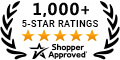The following problems involve the concept of Related Rates. In short, Related Rates problems combine word problems together with Implicit Differentiation, an application of the Chain Rule. Recall that if $y=f(x)$, then $D \{y \} = \displaystyle{ dy \over dx } = f'(x)=y'$. For example, implicitly differentiating the equation $$y^3+y^2= y+1$$ would be $$D\{y^3+y^2\} = D\{ y+1 \} \ \ \ \ \longrightarrow$$ $$3y^2 \cdot y'+ 2y \cdot y' = y' + 0$$ If $x=f(t)$ and $y=g(t)$, then $D\{x\} = \displaystyle{ dx \over dt } = f'(t)$ and $D\{y\} = \displaystyle{ dy \over dt } = g'(t)$ . For example, implicitly differentiating the equation $$x^3+y^2= x+y+3$$ would be $$D\{x^3+y^2\} = D\{ x+y+3 \} \ \ \ \ \longrightarrow$$ $$3x^2 \cdot \displaystyle{ dx \over dt } + 2y \cdot \displaystyle{ dy \over dt } = \displaystyle{ dx \over dt } + \displaystyle{ dy \over dt } + 0$$ In all of the following Related Rates Problems, it will be assumed that each variable function $y$ is a function of time $t$. For that reason, I will always use Leibniz notation and not the ambiguous prime notation for derivatives, i.e., i will use $$\displaystyle{ dy \over dt } \ \ \ \ instead \ of \ \ \ \ y'$$ Here is my strategy for approaching and solving Related Rates Problems: 1.) Read the problem slowly and carefully. 2.) Draw an appropriate sketch. 3.) Introduce and define appropriate variables. Use variables if quantities are changing. Use constants if quantities are not changing. 4.) Read the problem again. 5.) Clearly label the sketch using your variables. 6.) State what information is given in the problem. 7.) State what information is to be determined or found. 8.) Use a given equation or create an appropriate equation relating the given variables. 9.) Differentiate this equation with respect to the time variable $t$. 10.) Plug in the given rates and numbers to the differentiated equation. 11.) Solve for the unknown rate. 12.) Put proper units on your final answer. EXAMPLE 1: Consider a right triangle which is changing shape in the following way. The horizontal leg is increasing at the rate of $5 \ in./min.$ and the vertical leg is decreasing at the rate of $6 \ in./min$. At what rate is the hypotenuse changing when the horizontal leg is $12 \ in.$ and the vertical leg is $9 \ in.$ ? Draw a right triangle with legs labeled $x$ and $y$ and hypotenuse labeled $z$, and assume each edge is a function of time $t$. GIVEN: $\ \ \ \displaystyle{ dx \over dt } = 5 \ in./min. \$ and $\ \displaystyle{ dy \over dt }= -6 \ in./min.$ FIND: $\ \ \ \displaystyle{ dz \over dt }$ when $x=12 \ in.$ and $y=9 \ in$. Use the Pythagorean Theorem to get the equation $$x^2 + y^2 = z^2$$ Now differentiate this equation with repect to time $t$ getting $$D \{ x^2 + y^2\} = D \{z^2\} \ \ \ \longrightarrow$$ $$2x \displaystyle{ dx \over dt } + 2y \displaystyle{ dy \over dt } = 2z \displaystyle{ dz \over dt } \ \ \ \longrightarrow \ \$$ (Multiply both sides of the equation by $1/2$.) $$x \displaystyle{ dx \over dt } + y \displaystyle{ dy \over dt } = z \displaystyle{ dz \over dt } \ \ \ \ \ \ \ \ \ \ \ \ \ \ (DE)$$ Now let $x=12$ and $y=9$ and solve for $z$ using the Pythagorean Theorem. $$12^2+9^2= z^2 \ \ \ \longrightarrow \ \ \ z^2=225 \ \ \ \longrightarrow \ \ \ z=15$$ Plug in all given rates and values to the equation $(DE)$ getting $$(12)(5) + (9)(-6) = (15) \displaystyle{ dz \over dt } \ \ \ \longrightarrow$$ $$6 = 15 \displaystyle{ dz \over dt } \ \ \ \longrightarrow$$ $$\displaystyle{ dz \over dt } = {6 \over 15} = { 2 \over 5} \ in/min.$$ In the list of Related Rates Problems which follows, most problems are average and a few are somewhat challenging. PROBLEM 1 : The edge of a square is increasing at the rate of $\ 3 \ cm/sec$. At what rate is the square's $\ \ \ \$ a.) perimeter changing $\ \ \ \$ b.) area changing when the edge of the square is $10 \ cm.$ ? Click HERE to see a detailed solution to problem 1.

• Exponent Laws and Logarithm Laws
• Trig Formulas and Identities
• Differentiation Rules
• Trig Function Derivatives
• Table of Derivatives
• Table of Integrals
• Calculus Home

## 4 Steps to Solve Any Related Rates Problem – Part 1

• March 6, 2014
• by Bruce Birkett
• Tags: Calculus , ladder , problem solving strategy , related rates

Almost 90% of respondents find this blog post “very” or “extremely useful.” We hope you will too! If you’re working on a related rates problem and need help, please let us know on our Forum and we’ll do our best to assist.

Are you having trouble with Related Rates problems in Calculus? Let’s break ’em down and develop a strategy that you can use to solve them routinely for yourself.

## I. What’s related about these rates? (Or, “How to recognize a Related Rates problem.”)

Related rates problems will always give you the rate of one quantity that’s changing, and ask you to find the rate of something else that’s changing as a result. Here are three common problem-scenarios to illustrate:In each case you’re given the rate at which one quantity is changing. That is, you’re given the value of the derivative with respect to time of that quantity:

• “The radius . . . increases at 1 millimeter each second” means the radius changes at the rate of  $\dfrac{dr}{dt} = 1$ mm/s.
• “The base of the ladder slides horizontally away from the wall at 2 feet per second” means the ladder-base’s x -position changes at the rate of  $\dfrac{dx}{dt} = 2$ ft/s.
• When a quantity is decreasing, we have to make the rate negative. “A cone is being drained of water at the constant rate of 15 cm$^3$ each second” means the volume of water in cone changes at the rate of  $\dfrac{dV}{dt} = -15$ cm$^3$/s. Notice that we have to make the rate negative to capture that the water’s volume is decreasing . To do so, we insert the negative sign “by hand”—we just stick it in there.

The upshot: Related rates problems will always tell you about the rate at which one quantity is changing (or maybe the rates at which two quantities are changing), often in units of distance/time, area/time, or volume/time. The question will then be \left. \begin{align*} \text{how fast. . . } \\ \text{how quickly. . . } \\ \text{at what rate. . .}\\ \end{align*} \right\} $$\text{…does another quantity change as a result?}$$ The rate you’re after is related to the rate(s) you’re given. Your job is to find that relationship. RELATED MATERIAL

• Related Rates Problems & Complete Solutions
• “ How to Solve Optimization Problems”

## II. Your Job: Develop a Relationship between Quantities

• the quantity you’re after, and
• the quantity (or quantities) you’ve been given.

You’ll take a derivative in a minute to get a relationship between the rates . Before then, though, we have to write a relationship between the quantities themselves. Fortunately, there’s just a tiny handful of approaches you’ll ever use. We’ll illustrate two of the most common using our first two examples above. (We’ll analyze the third example in our next post .)

• the quantity you’re after (something about the circle’s area, A ), and
• the quantity you’ve been given (something about the circle’s radius, r ).• the quantity you’re after (something about the ladder-top’s vertical position, y ), and
• the quantity you’ve been given (something about the ladder-bottom’s horizontal position, x ).

The upshot: The new skill you’ll probably need to practice is developing the relationship between the quantity whose rate you’re after, and the quantity (or quantities) whose rate(s) you know about. There are only a few approaches you’ll typically use; we’ve listed them all in our Problem Solving Strategy box below.

☕ Buy us a coffee We're working to add more, and would appreciate your help to keep going! 😊

## III. Take the Derivative with Respect to Time

Related Rates questions always ask about how two (or more) rates are related, so you’ll always take the derivative of the equation you’ve developed with respect to time . That is, take $\dfrac{d}{dt}$ of both sides of your equation. Be sure to remember the Chain Rule!

Let’s apply this step to the equations we developed in our two examples above:

1. Expanding Circle Example. \begin{align*} A &= \pi r^2 \\ \\ \frac{d}{dt}[A] &= \frac{d}{dt}\left[\pi r^2 \right] \\ \\ \frac{dA}{dt} &= 2\pi r \frac{dr}{dt} \end{align*}

While the derivative of $r^2$ with respect to r is $\dfrac{d}{dr}r^2 = 2r$, the derivative of $r^2$ with respect to time t is $\dfrac{d}{dt}r^2 = 2r\dfrac{dr}{dt}$.

Remember that r is a function of time t : the radius grows as time passes. We could have captured this time-dependence explicitly by writing our relation as $$A(t) = \pi [r(t)]^2$$ to remind ourselves that both A and r are functions of time t . Then when we take the derivative, \begin{align*} \frac{dA(t)}{dt} &= 2\pi r(t) \left[\frac{d}{dt}r(t)\right]\\ \\ &= 2\pi r(t) \left[\frac{dr}{dt}\right] \end{align*}

Most people find that writing the explicit time-dependence A(t) and r(t) annoying, and so just write A and r instead. Regardless, you must remember that r depends on t , and so when you take the derivative with respect to time the Chain Rule applies and you have the $\dfrac{dr}{dt}$ term. [collapse] That last expression gives us the relation we need between the unknown $\dfrac{dA}{dt}$ and the known $\dfrac{dr}{dt} = 1 \,\frac{\text{mm}}{\text{s}}$, and so we’re essentially finished. The problem will always specify the other conditions of the moment you’re interested in. For instance, this problem might have asked:

Question: How fast is the circle’s area changing when its radius is 20 mm?The problem could have specified any value for r ; it doesn’t matter. At this point, we’re just substituting in values.

2. Sliding Ladder Example. \begin{align*} x^2 + y^2 &= 10^2 \\ \\ \frac{d}{dt}\left[ x^2 + y^2\right] &= \frac{d}{dt}[10^2] \\ \\ 2x\frac{dx}{dt} + 2y\frac{dy}{dt} &= 0 \text{ [*]} \\ \end{align*}

The last expression gives us the relation we need between the unknown $\dfrac{dy}{dt}$ and the known $\dfrac{dx}{dt} = 2 \,\frac{\text{ft}}{\text{s}}$, so again the problem is essentially finished. If it had asked, for instance,

Question: How quickly is the top of the ladder sliding down the wall when the base is 8 feet from the wall?

then we would have x = 8 ft and $\dfrac{dx}{dt} = 2$ ft/s, and we’re looking for $\dfrac{dy}{dt}$. But then in this case we still have one unknown: we don’t know y .

So we have to stop and do a quick subproblem: our original Pythagorean statement specifies y when $x = 8$.

• Begin subproblem to find $y$ when $x = 8$:

\begin{align*} x^2 + y^2 &= 10^2 \\ 8^2 + y^2 &= 100 \\ y^2 &= 100 – 64 = 36 \\ y &= 6 \text{ ft} \\ \end{align*} End subproblem.

So now we have $x =8$ ft, $y = 6$ ft, and $\dfrac{dx}{dt} = 2$ ft/s. We’re solving for $\dfrac{dy}{dt}$.Starting with the equation marked [*] above: \begin{align*}2x\frac{dx}{dt} + 2y\frac{dy}{dt} &= 0 \\[8px] 2y\frac{dy}{dt} &= -2x\frac{dx}{dt} \\[8px] \frac{dy}{dt} &= -\frac{x}{y}\frac{dx}{dt} \\[8px] &= – \frac{8}{6}(2)\\[8px] &= -\frac{8}{3}\,\frac{\text{ft}}{\text{s}} \quad \cmark \end{align*}

Again, the problem could have given us any value for x, and then we’d find the associated y and substitute those values in. (Or the problem could specify a value for y and then we’d have to find the associated x . Whatever.) At this point we’re just substituting in values.

3. Water Leaving a Cone Example. To see the complete solution to this problem, please visit Part 2 of this blog post on how to solve related rates problems.

## Quick links: Related Rates Problems & Solutions

• Snowball melts
• Rate angle changes as a ladder slides away from a house
• Water level falls as it drains from a cone
• Lamp post casts a shadow of a man walking
• Given an equation with x and y , find a rate

## IV. 4-Step Problem Solving StrategyAs promised, in the next post we’ll complete the “Water Leaving A Cone” example, which will illustrate the common use of similar triangles in solving Related Rates problems.

☕ Buy us a coffee If we've helped, please consider giving a little something back. Thank you! 😊

Over to you:

• What tips do you have to share about solving Related Rates problems?
• Or what questions do you have? Related rates problems can be tricky to start, and we’re happy to help !
• Or how can we make posts such as this one more useful to you?

Please post on the Forum and let us know!

Share a link to this screen:

• Share on Pinterest
• Share on Reddit
• Share on Telegram
• Share on WhatsApp
• Share on SMS

## What are your thoughts or questions?

Thank u sir

You are very welcome. We are happy to have helped!

Really helpful, i never understood related rates before seeing this site

Thanks so much for letting us know. We’re very happy to have helped! : )

It was really helpful and interesting,i like it

Thank you very much….

You are very welcome! : )

I wish my AP Calculus teacher could explain things clearly. I am going to let her know about this blog.

Thanks, Rebecca! We’re happy to have helped.

## Latest Blog Posts

• Function with Given Properties – Problem of the Week
• Differentiability & Continuity – Problem of the Week
• Discontinuities & Asymptotes – Problem of the Week

• Evaluating Limits
• Limits at Infinity
• Limits at Infinity with Square Roots
• Calculating Derivatives
• Equation of a Tangent Line
• Mean Value Theorem & Rolle’s Theorem
• Garden fence
• Least expensive open-topped can
• Printed poster
• Snowball melts, area decreases at given rate
• How fast is the ladder’s top sliding
• Angle changes as a ladder slides
• Lamp post casts shadow of man walking
• Water drains from a cone
• Given an equation, find a rate

Have a question you could use some help with? Please post on our Forum:

Community.Matheno.com

It's free for your use, just to support your learning. We'd love to help!

## This site is free?!?

We don't charge for anything on this site, we don't run ads, and we will never sell your personal information.

We're passionate educators with a goal:

Provide high-quality, interactive materials to dedicated learners everywhere in the world, regardless of ability to pay, so they (you!) can learn well and excel.Matheno ®

Berkeley, CaliforniaAP ® is a trademark registered by the College Board, which is not affiliated with, and does not endorse, this site.

• Your self-chosen confidence rating for each problem, so you know which to return to before an exam (super useful!);
• Your progress, and specifically which topics you have marked as complete for yourself.

Your selections are for your use only, and we do not share your specific data with anyone else. We do use aggregated data to help us see, for instance, where many students are having difficulty, so we know where to focus our efforts.

You will also be able to post any Calculus questions that you have on our Forum , and we'll do our best to answer them!

We believe that free, high-quality educational materials should be available to everyone working to learn well. There is no cost to you for having an account, other than our gentle request that you contribute what you can, if possible, to help us maintain and grow this site.

You drag the end of a 10 feet ladder along the ground at a rate of 2 ft/s.

How fast is its top along a wall descending as a function of time, given it started vertical at t � =0?

x 2 + y 2 = 100, x = 2t until t = 5.

2xdx + 2ydy = 0

which gives the chain rule:

## Module 4: Applications of Derivatives

Related-rates problem-solving, learning outcomes.

• Express changing quantities in terms of derivatives.
• Find relationships among the derivatives in a given problem.
• Use the chain rule to find the rate of change of one quantity that depends on the rate of change of other quantities.

## Setting up Related-Rates Problems

In many real-world applications, related quantities are changing with respect to time. For example, if we consider the balloon example again, we can say that the rate of change in the volume, $V$, is related to the rate of change in the radius, $r$. In this case, we say that $\frac{dV}{dt}$ and $\frac{dr}{dt}$ are related rates because $V$ is related to $r$. Here we study several examples of related quantities that are changing with respect to time and we look at how to calculate one rate of change given another rate of change.

## Example: Inflating a Balloon

A spherical balloon is being filled with air at the constant rate of $2 \, \frac{\text{cm}^3}{\text{sec}}$ (Figure 1). How fast is the radius increasing when the radius is $3\, \text{cm}$?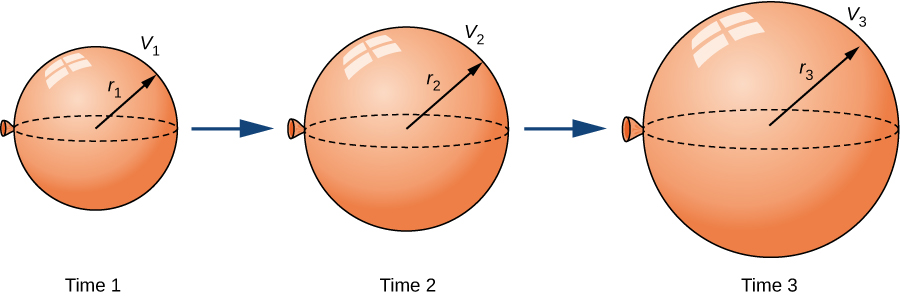Figure 1. As the balloon is being filled with air, both the radius and the volume are increasing with respect to time.

The volume of a sphere of radius $r$ centimeters is

Since the balloon is being filled with air, both the volume and the radius are functions of time. Therefore, $t$ seconds after beginning to fill the balloon with air, the volume of air in the balloon is

Differentiating both sides of this equation with respect to time and applying the chain rule, we see that the rate of change in the volume is related to the rate of change in the radius by the equation

The balloon is being filled with air at the constant rate of 2 cm 3 /sec, so $V^{\prime}(t)=2 \, \text{cm}^3 / \sec$. Therefore,

which implies

When the radius $r=3 \, \text{cm}$,

Watch the following video to see the worked solution to Example: Inflating a Balloon.

What is the instantaneous rate of change of the radius when $r=6 \, \text{cm}$?

$\frac{dr}{dt}=\dfrac{1}{2\pi r^2}$

$\dfrac{1}{72\pi} \, \text{cm/sec}$, or approximately 0.0044 cm/sec

Before looking at other examples, let’s outline the problem-solving strategy we will be using to solve related-rates problems.

## Problem-Solving Strategy: Solving a Related-Rates Problem

• Assign symbols to all variables involved in the problem. Draw a figure if applicable.
• State, in terms of the variables, the information that is given and the rate to be determined.
• Find an equation relating the variables introduced in step 1.
• Using the chain rule, differentiate both sides of the equation found in step 3 with respect to the independent variable. This new equation will relate the derivatives.
• Substitute all known values into the equation from step 4, then solve for the unknown rate of change.

We are able to solve related-rates problems using a similar approach to implicit differentiation. In the example below, we are required to take derivatives of different variables with respect to time ${t}$, ie. ${s}$ and ${x}$. When this happens, we can attach a $\frac{ds}{dt}$ or a $\frac{dx}{dt}$ to the derivative, just as we did in implicit differentiation.

Note that when solving a related-rates problem, it is crucial not to substitute known values too soon. For example, if the value for a changing quantity is substituted into an equation before both sides of the equation are differentiated, then that quantity will behave as a constant and its derivative will not appear in the new equation found in step 4. We examine this potential error in the following example.

## Examples of the Process

Let’s now implement the strategy just described to solve several related-rates problems. The first example involves a plane flying overhead. The relationship we are studying is between the speed of the plane and the rate at which the distance between the plane and a person on the ground is changing.

## Example: An Airplane Flying at a Constant Elevation

An airplane is flying overhead at a constant elevation of $4000$ ft. A man is viewing the plane from a position $3000$ ft from the base of a radio tower. The airplane is flying horizontally away from the man. If the plane is flying at the rate of $600$ ft/sec, at what rate is the distance between the man and the plane increasing when the plane passes over the radio tower?

Step 1. Draw a picture, introducing variables to represent the different quantities involved.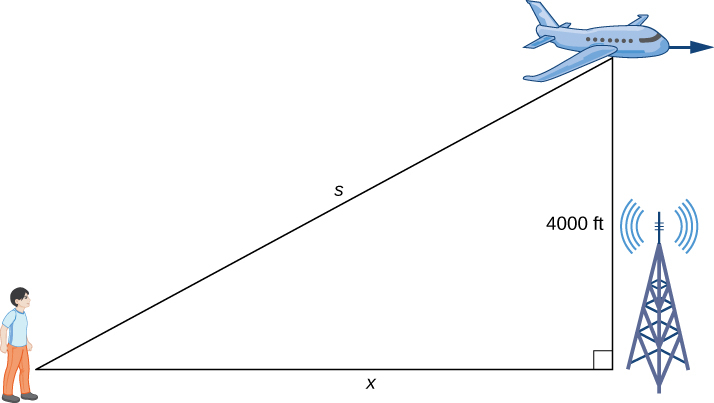Figure 2. An airplane is flying at a constant height of 4000 ft. The distance between the person and the airplane and the person and the place on the ground directly below the airplane are changing. We denote those quantities with the variables $s$ and $x$, respectively.

As shown, $x$ denotes the distance between the man and the position on the ground directly below the airplane. The variable $s$ denotes the distance between the man and the plane. Note that both $x$ and $s$ are functions of time. We do not introduce a variable for the height of the plane because it remains at a constant elevation of $4000$ ft. Since an object’s height above the ground is measured as the shortest distance between the object and the ground, the line segment of length $4000$ ft is perpendicular to the line segment of length $x$ feet, creating a right triangle.

Step 2. Since $x$ denotes the horizontal distance between the man and the point on the ground below the plane, $dx/dt$ represents the speed of the plane. We are told the speed of the plane is 600 ft/sec. Therefore, $\frac{dx}{dt}=600$ ft/sec. Since we are asked to find the rate of change in the distance between the man and the plane when the plane is directly above the radio tower, we need to find $ds/dt$ when $x=3000$ ft.

Step 3. From Figure 2, we can use the Pythagorean theorem to write an equation relating $x$ and $s$:

$[x(t)]^2+4000^2=[s(t)]^2$.

Step 4. Differentiating this equation with respect to time and using the fact that the derivative of a constant is zero, we arrive at the equation

$x\frac{dx}{dt}=s\frac{ds}{dt}$.

Step 5. Find the rate at which the distance between the man and the plane is increasing when the plane is directly over the radio tower. That is, find $\frac{ds}{dt}$ when $x=3000$ ft. Since the speed of the plane is $600$ ft/sec, we know that $\frac{dx}{dt}=600$ ft/sec. We are not given an explicit value for $s$; however, since we are trying to find $\frac{ds}{dt}$ when $x=3000$ ft, we can use the Pythagorean theorem to determine the distance $s$ when $x=3000$ and the height is $4000$ ft. Solving the equation

for $s$, we have $s=5000$ ft at the time of interest. Using these values, we conclude that $ds/dt$ is a solution of the equation

$\frac{ds}{dt}=\frac{3000 \cdot 600}{5000}=360$ ft/sec.

Note : When solving related-rates problems, it is important not to substitute values for the variables too soon. For example, in step 3, we related the variable quantities $x(t)$ and $s(t)$ by the equation

Since the plane remains at a constant height, it is not necessary to introduce a variable for the height, and we are allowed to use the constant 4000 to denote that quantity. However, the other two quantities are changing. If we mistakenly substituted $x(t)=3000$ into the equation before differentiating, our equation would have been

After differentiating, our equation would become

As a result, we would incorrectly conclude that $\frac{ds}{dt}=0$.

Watch the following video to see the worked solution to Example: An Airplane Flying at a Constant Elevation.

What is the speed of the plane if the distance between the person and the plane is increasing at the rate of $300$ ft/sec?

$\frac{ds}{dt}=300$ ft/sec

$500$ ft/sec

What rate of change is necessary for the elevation angle of the camera if the camera is placed on the ground at a distance of $4000$ ft from the launch pad and the velocity of the rocket is $500$ ft/sec when the rocket is $2000$ ft off the ground?

Find $\frac{d\theta}{dt}$ when $h=2000$ ft. At that time, $\frac{dh}{dt}=500$ ft/sec.

$\frac{1}{10}$ rad/sec

In the next example, we consider water draining from a cone-shaped funnel. We compare the rate at which the level of water in the cone is decreasing with the rate at which the volume of water is decreasing.

## Example: Water Draining from a Funnel

Water is draining from the bottom of a cone-shaped funnel at the rate of $0.03 \, \text{ft}^3 /\text{sec}$. The height of the funnel is $2$ ft and the radius at the top of the funnel is $1$ ft. At what rate is the height of the water in the funnel changing when the height of the water is $\frac{1}{2}$ ft?

Step 1: Draw a picture introducing the variables.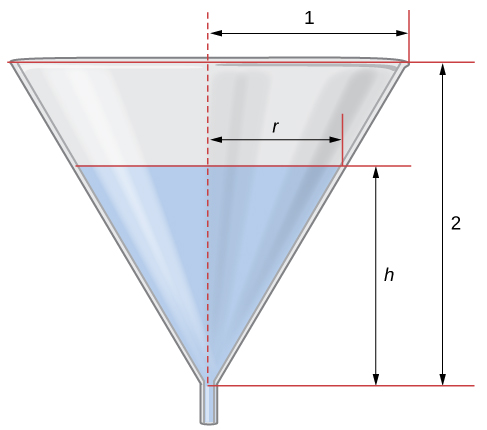Figure 3. Water is draining from a funnel of height 2 ft and radius 1 ft. The height of the water and the radius of water are changing over time. We denote these quantities with the variables $h$ and $r,$ respectively.

Let $h$ denote the height of the water in the funnel, $r$ denote the radius of the water at its surface, and $V$ denote the volume of the water.

Step 2: We need to determine $\frac{dh}{dt}$ when $h=\frac{1}{2}$ ft. We know that $\frac{dV}{dt}=-0.03 \text{ft}^3 / \text{sec}$.

Step 3: The volume of water in the cone is

From Figure 3, we see that we have similar triangles. Therefore, the ratio of the sides in the two triangles is the same. Therefore, $\frac{r}{h}=\frac{1}{2}$ or $r=\frac{h}{2}$. Using this fact, the equation for volume can be simplified to

$V=\frac{1}{3}\pi (\frac{h}{2})^2 h=\frac{\pi}{12}h^3$

Step 4: Applying the chain rule while differentiating both sides of this equation with respect to time $t$, we obtain

$\frac{dV}{dt}=\frac{\pi}{4}h^2 \frac{dh}{dt}$

Step 5: We want to find $\frac{dh}{dt}$ when $h=\frac{1}{2}$ ft. Since water is leaving at the rate of $0.03 \, \text{ft}^3 / \text{sec}$, we know that $\frac{dV}{dt}=-0.03 \, \text{ft}^3 / \text{sec}$. Therefore,

It follows that

At what rate is the height of the water changing when the height of the water is $\frac{1}{4}$ ft?

$-0.61$ ft/sec

We need to find $\frac{dh}{dt}$ when $h=\frac{1}{4}$.

• 4.1 Related Rates. Authored by : Ryan Melton. License : CC BY: Attribution
• Calculus Volume 1. Authored by : Gilbert Strang, Edwin (Jed) Herman. Provided by : OpenStax. Located at : https://openstax.org/details/books/calculus-volume-1 . License : CC BY-NC-SA: Attribution-NonCommercial-ShareAlike . License Terms : Access for free at https://openstax.org/books/calculus-volume-1/pages/1-introduction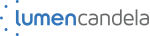#### IMAGES

1. 4 Steps to Solve Any Related Rates Problem2. Lesson 2.12A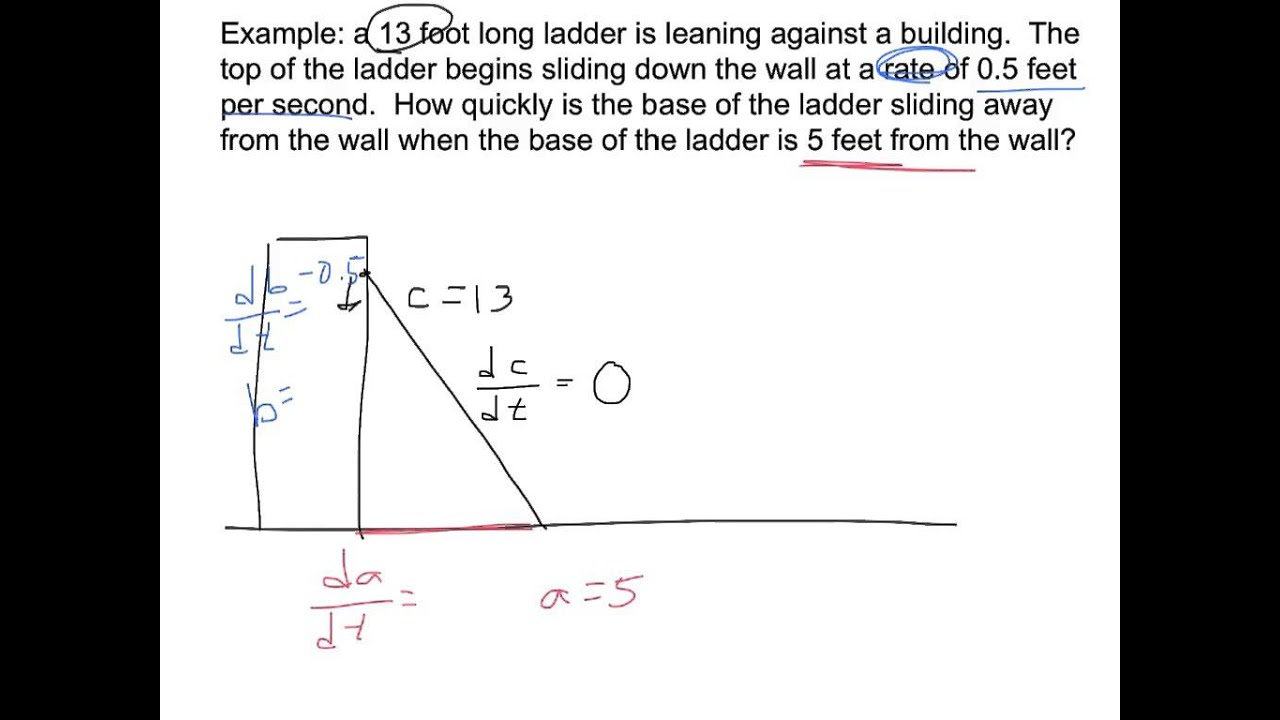3. Related Rates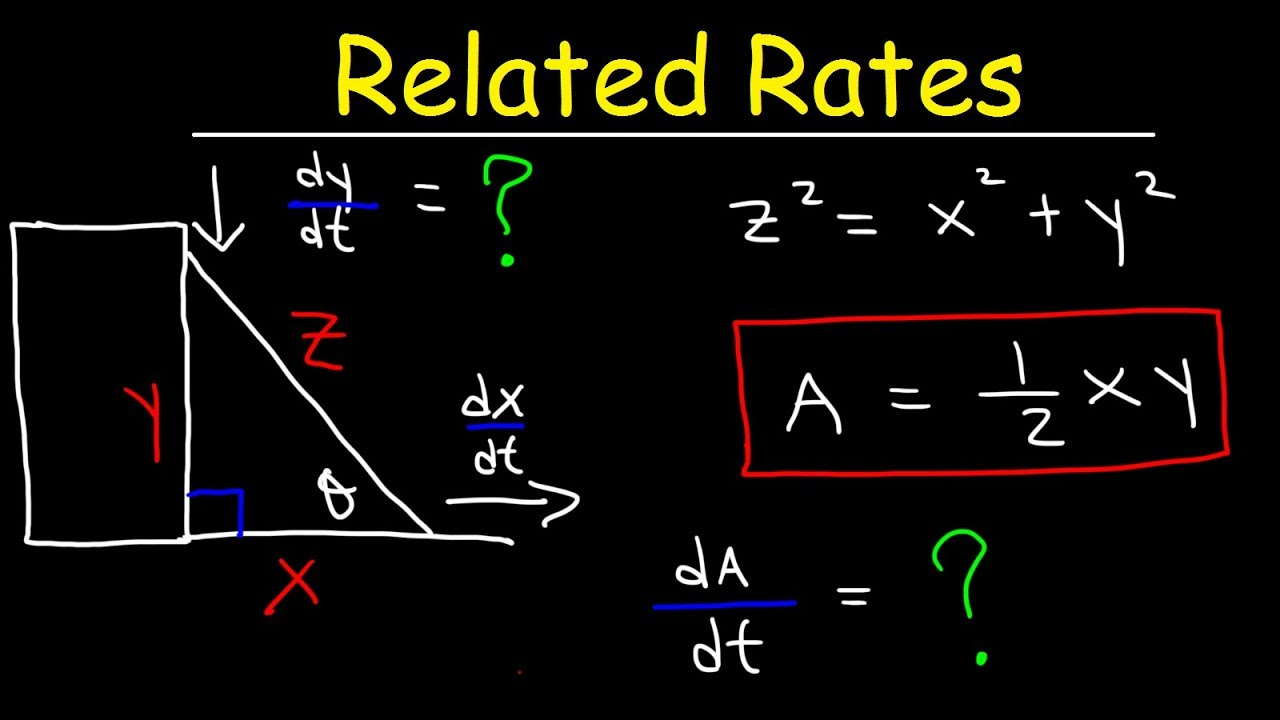4. Related Rates: The Ladder Problem || Calculus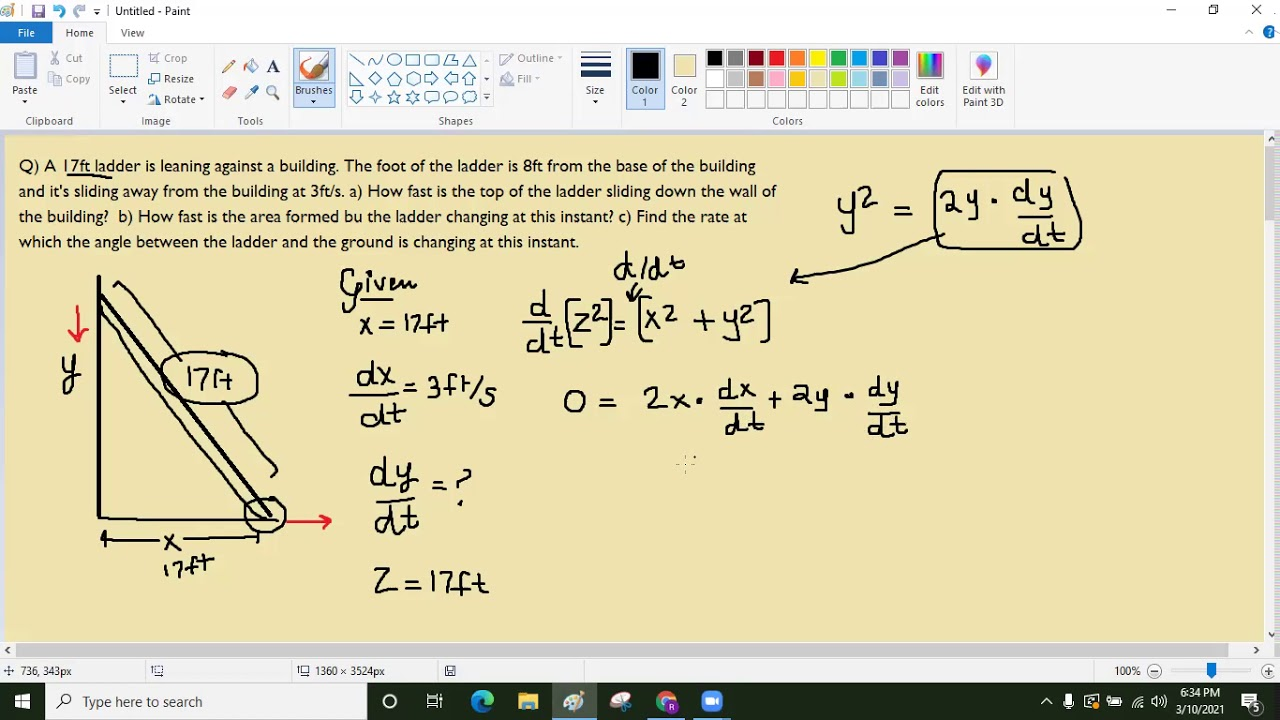5. Solving Related Rates Problems in Calculus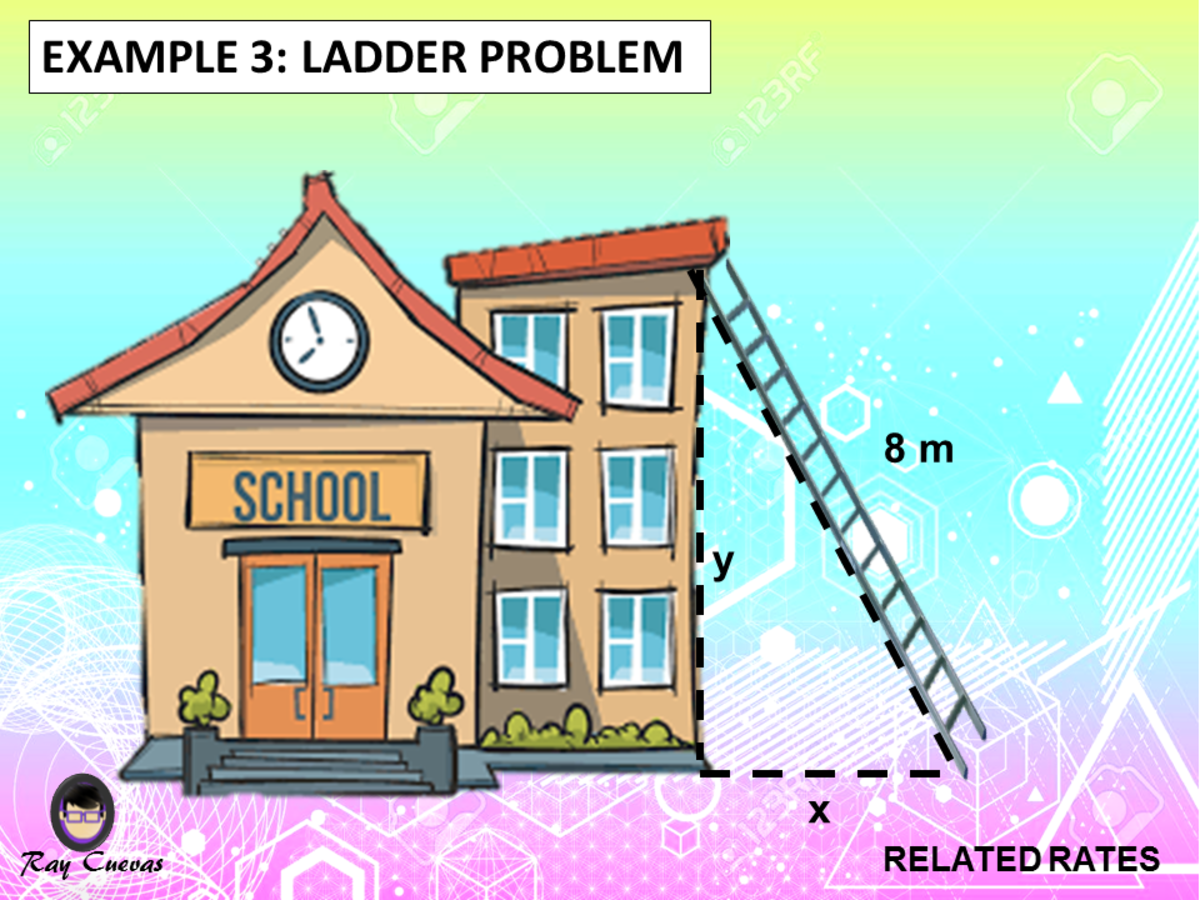6. Ex 4: Related Rates: Ladder Problem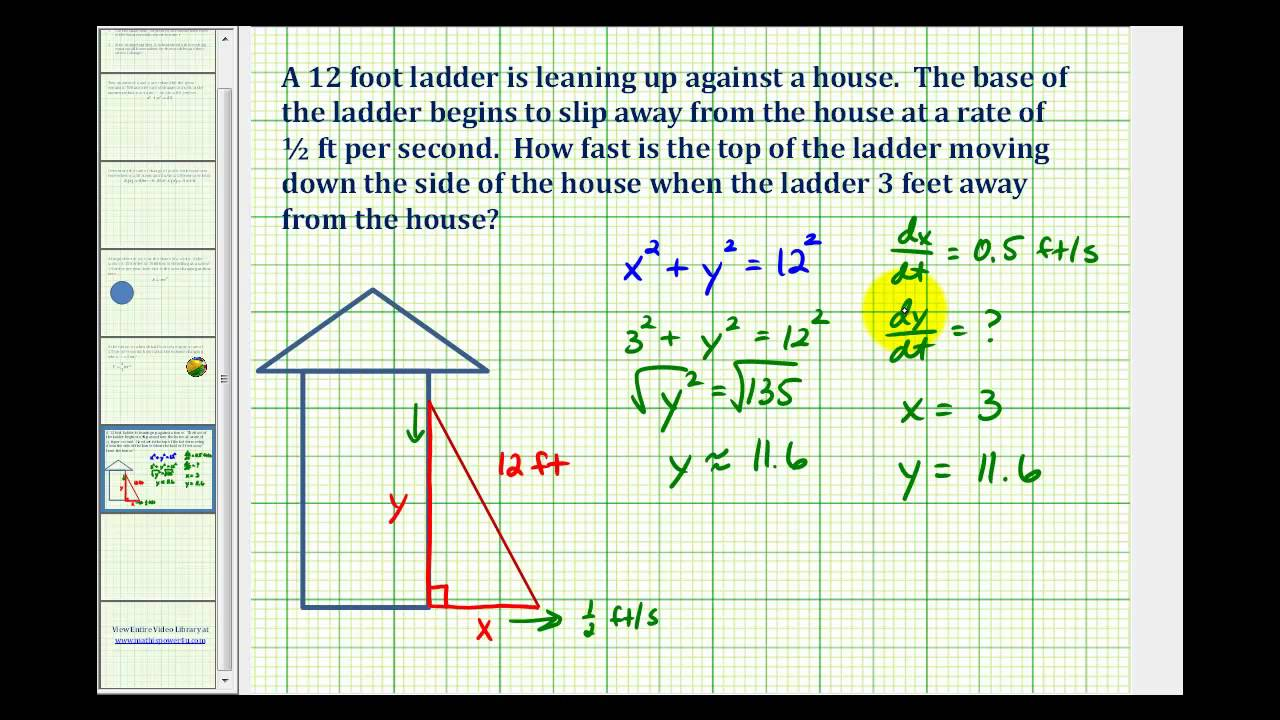1. how fast is the ladder's top sliding (related rates problem)

Calculus Solution Related rates - Ladder slides (Matheno.com) Watch on To solve this problem, we will use our standard 4-step Related Rates Problem Solving Strategy. 1. Draw a picture of the physical situation. See the figure. Let x be the horizontal distance, in feet, from the wall to the bottom of the ladder.

2. Related rates: Falling ladder (video)

Cyan Wind 9 years ago Did Sal use implicit differentiation in this example because there is a relationship between x and h (x² + h² = 100)? If yes, can we change it to h = sqrt (100 - x²) and calculate d/dt (sqrt (100 - x²)) instead? • ( 10 votes) Upvote Flag neal 9 years ago

3. Related Rates

This calculus video tutorial explains how to solve the ladder problem in related rates. It explains how to find the rate at which the top of the ladder is s...

1. Draw a sketch As always, we'll start by drawing a quick sketch of all of the information that is being described in the problem. To do this we should first think about what information we have. First of all, we need to think about the shape that's being formed with the ladder.

5. 4.1 Related Rates

Problem-Solving Strategy: Solving a Related-Rates Problem Assign symbols to all variables involved in the problem. Draw a figure if applicable. State, in terms of the variables, the information that is given and the rate to be determined. Find an equation relating the variables introduced in step 1.

6. Related Rates Ladder Problem (Calculus)

Learn how to solve Calculus Related Rate problems specifically the ladder sliding down the wall in this free math video tutorial by Mario's Math Tutoring. W...

This MATHguide math education video demonstrates how to solve a related rates problem -- a ladder problem. This problem uses the chain rule and sum rule for... This MATHguide math...

8. Analyzing problems involving related rates

Related rates problems are applied problems where we find the rate at which one quantity is changing by relating it to other quantities whose rates are known. Worked example of solving a related rates problem Imagine we are given the following problem: The radius r ( t) of a circle is increasing at a rate of 3 centimeters per second.

9. Related rates intro (practice)

Lesson 5: Solving related rates problems. Related rates intro. ... Related rates: Falling ladder. Related rates (Pythagorean theorem) Related rates: water pouring into a cone. Related rates (advanced) Related rates: shadow. Related rates: balloon. Math > AP®︎/College Calculus AB > Contextual applications of differentiation >

10. Related rates (multiple rates) (practice)

Related rates (multiple rates) Google Classroom. You might need: Calculator. The base of a triangle is decreasing at a rate of 13 millimeters per minute and the height of the triangle is increasing at a rate of 6 millimeters per minute. At a certain instant, the base is 5 millimeters and the height is 1 millimeter.

11. Related Rates (How To w/ 7+ Step-by-Step Examples!)

How To Solve Related Rates Problems We use the principles of problem-solving when solving related rates. The steps are as follows: Read the problem carefully and write down all the given information. Sketch and label a graph or diagram, if applicable.

12. Related Rates

Related Rates - Ladder. Save Copy. Log InorSign Up. Problem Version #81517. 1. A 17-ft ladder is leaning against a barn when its base starts to slide away at a constant rate of 1 ft/sec. How fast is the top of the ladder sliding down the barn wall when the base of the ladder is 8 ft from the barn wall along the ground?

13. calculus

0. First when you use implicit to differentiate x2 +y2 =z2. You get 2xdx dt + 2ydy dt = 2zdz dt. But since dz dt means the rate at which that side changes, but since the ladder won't change size because its a ladder. So dz dt is 0, so when you plug it in you get 2xdx dt + 2ydy dt = 2(25)(0) which turns to 0.

14. Angle change as a ladder slides (related rates problem)

Calculus Solution To solve this problem, we will use our standard 4-step Related Rates Problem Solving Strategy. 1. Draw a picture of the physical situation. See the figure. We've labeled the angle that the ladder makes with the ground, since the problem is asking us to find the rate at which that angle changes, , at a particular moment — when .

15. Solving Related Rates Problems

1.) Read the problem slowly and carefully. 2.) Draw an appropriate sketch. 3.) Introduce and define appropriate variables. Use variables if quantities are changing. Use constants if quantities are not changing. 4.) Read the problem again. 5.) Clearly label the sketch using your variables. 6.) State what information is given in the problem. 7.)

16. 4 Steps to Solve Any Related Rates Problem

4 Steps to Solve Any Related Rates Problem - Part 1 March 6, 2014 by Bruce Birkett Tags: Calculus, ladder, problem solving strategy, related rates 28 Comments Almost 90% of respondents find this blog post "very" or "extremely useful." We hope you will too!

You drag the end of a 10 feet ladder along the ground at a rate of 2 ft/s. How fast is its top along a wall descending as a function of time, given it started vertical at t =0? x 2 + y 2 = 100, x = 2t until t = 5. 2xdx + 2ydy = 0. which gives the chain rule:

18. Related Rates

This calculus video tutorial explains how to solve related rates problems using derivatives. It shows you how to calculate the rate of change with respect to radius, height, surface area,...

19. RELATED RATES LADDER PROBLEM EXAMPLE

See how to solve this related rates ladder problem example with 4 simple steps. I'll walk you through how to apply these 4 steps that you can use for any re...

20. Related-Rates Problem-Solving

Problem-Solving Strategy: Solving a Related-Rates Problem. Assign symbols to all variables involved in the problem. Draw a figure if applicable. State, in terms of the variables, the information that is given and the rate to be determined. Find an equation relating the variables introduced in step 1.

21. Calculus I

Example 1 Air is being pumped into a spherical balloon at a rate of 5 cm 3 /min. Determine the rate at which the radius of the balloon is increasing when the diameter of the balloon is 20 cm. Show Solution We can get the units of the derivative by recalling that, r′ = dr dt r ′ = d r d t

22. Solving Related Rates Problems in Calculus

Ray Updated: Jul 22, 2022 9:36 AM EDT Photo by Shubham Sharan on Unsplash What Are Related Rates? Related Rates are calculus problems that involve finding a rate at which a quantity changes by relating to other known values whose rates of change are known.